Latest Banking jobs   »   Reasoning Ability Quiz For Bank Mains...

# Reasoning Ability Quiz For Bank Mains Exams 2021- 6th February

Direction (1-5): Study the following information carefully to answer the given question.
Eight persons-K, C, R, I. E, O, M and S are sitting around a circular table, but not necessarily in the same order. Four of them are facing inside and the other four are facing outside. All eight persons have different ages. I sits third to the right of C, who is one year older than the person who sits to the immediate left of E. The person who is sitting second to the left of I is facing the same direction as the person who is Forty-Nine years old. Immediate neighbours of E are Seventeen and Twenty years old. K is Forty-Nine years old. O is Fifty-four years old. E sits to the immediate left of M. The person who is Twenty-five years old is facing outwards and is an immediate neighbour of the person who is Twenty years old. S is an immediate neighbour of the persons who are Forty-Nine and Twenty-five years old. R faces the centre and sits third to the right of O. M is seventeen years old and faces the person who is Thirty years old. Age of E is equal to the number obtained by reversing the digits of C’s age. E is not the youngest. K does not sit second to the left of I.

Q1. Who among the following is the oldest?
(a) K
(b) M
(c) S
(d) I
(e) E

Q2. Who among the following are the immediate neighbours of the person whose age is three times that of C?
(a) C and O
(b) E and M
(c) S and C
(d) I and E
(e) M and C

Q3. What is the sum of the ages of S, I and R?
(a) 60
(b) 70
(c) 75
(d) 101
(e) None of these

Q4. What is the position of C with respect to E?
(a) Third to the right
(b) Fourth to the left
(c) Sixth to the right
(d) Second to the right
(e) Third to the left

Q5. Who among the following is sitting sixth to the right of the person who is twenty-five years old?
(a) O
(b) M
(c) R
(d) S
(e) E

Directions (6-7): Following questions are based on the information given below.
‘P × Q’ means ‘P is father of Q’
‘P – Q’ means ‘P is sister of Q’
‘P + Q’ means ‘P is mother of Q’
‘P ÷ Q’ means ‘P is the brother of Q’

Q6. In the expression A × R –G + D ÷ N, how is D related to A?
(a) Granddaughter
(b) Son
(c) Grandson
(d) Granddaughter or Grandson
(e) None of these

Q7. Which of the following represents ‘Q’ is son of ‘U’?
(a) Q ÷ I – G × U
(b) Q + I – G × U
(c) Q ÷ M – N × U
(d) Q ÷ J – N + U
(e) None of these

Directions (8-9): Following questions are based on the information given below.
‘P × Q’ means ‘P is Son of Q’
‘P – Q’ means ‘P is sister of Q’
‘P + Q’ means ‘P is mother of Q’
‘P ÷ Q’ means ‘P is the brother of Q’

Q8. In the expression R ÷ Q + M ÷ N × G, how is N related to R?
(a) Granddaughter
(b) Son
(c) Niece
(d) Granddaughter or Grandson
(e) None of these

Q9. In which of the following expression Q is wife of Z?
(a) A–M÷Q+N×Z
(b) A–Q÷M+N×Z
(c) Q–M÷A+N×Z
(d) Q–R÷A÷N×Z
(e) None of these

Directions (10): Study the following information carefully and answer the questions that follow:
A ÷ B means A is son of B
A × B means A is sister of B
A @ B means A is brother of B
A – B means A is mother of B

Q10. How is D related to A in the expression ‘A × R @ D – H’?
(a) Sister
(b) Daughter
(c) Son
(d) Mother
(e) None of these

Directions: (11-12): In the following questions, the symbols @, \$, #, © and % are used with the following meaning as illustrated below.
‘P \$ Q’ means ‘P is not smaller than Q’.
‘P © Q’ means ‘P is neither greater than nor equal to Q’.
‘P # Q’ means ‘P is neither smaller than nor equal to Q’.
‘P % Q’ means ‘P is not greater than Q’.
‘P @ Q’ means ‘P is neither greater than nor smaller than Q’.
Now, in each of the following questions, assuming the given statements to be true, find which of the four conclusions I, II, III and IV given below them is/are definitely true and give your answer accordingly.

Q11. Statements: R # J, J \$ D, D @ K, K % T
Conclusions:
I. T # D
II. T @ D
III. R # K
IV. J \$ T
(a) Only either I or II is true
(b) Only III is true
(c) Only III and IV are true
(d) Only III and either I or II are true
(e) None of these

Q12. Statements: T % R, R \$ M, M @ D, D © H
Conclusions:
I. D % R
II. H # R
IV. T % D
(a) Only I is true
(b) Only I and IV are true
(c) Only I and III are true
(d) Only II and IV are true
(e) None of these

Directions (13-15): Study the following information carefully and answer the questions given below:
P is 16m North of Q.T is 12m east of Q. O is midpoint between Q and T.R is 8m north of O. S is 6m west of R. U is 20m south of T. V is in the south of Q and to the west of U. J is in the north of R and east of P. K is the north of T.

Q13. What is the shortest distance between S and J?
(a) 10m
(b) √10m
(c) 14m
(d) 16m
(e) Cannot be determined

Q14. S is in which direction with respect to K?
(a) South-west
(b) West
(c) North-east
(d) North West
(e) Cannot be determined

Q15. If L is midpoint between U and V what is the shortest distance between J and L?
(a) 30m
(b) 35m
(c) 32m
(d) 36m
(e) None of these

Practice More Questions of Reasoning for Competitive Exams:

###### Bank Mains Cracker Study Plan 2021 : Check Now

Solutions

Solutions (1-5):
Sol.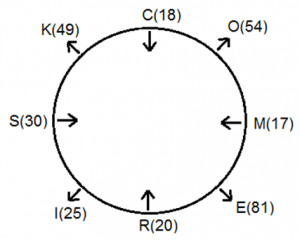S1. Ans.(e)
S2. Ans.(e)
S3. Ans.(c)
S4. Ans.(e)
S5. Ans.(e)

S6. Ans. (c)
Sol.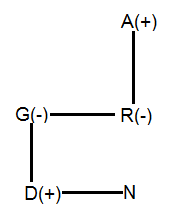S7. Ans. (e)

S8.Ans.(e)
Sol. After de-coding the given coded blood relation we get the relation between N and R in which N is the nephew of R.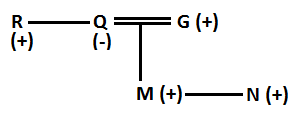S9.Ans.(a)
Sol. From Expression 1 we get our final answer and deduce the blood relation given below: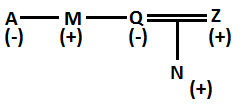S10.Ans.(a)
Sol. D is the sister of A.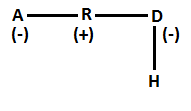Solutions (11-12):
Sol.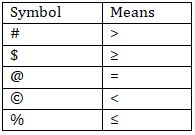S11.Ans.(d)
Sol. Statement= R >J ≥ D = K ≤ T

S12.Ans.(a)
Sol. Statement= T ≤ R ≥ M = D < H

Solutions (13-15):

Sol.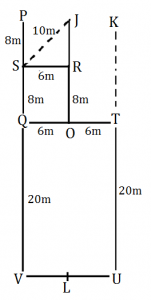S13. Ans. (a)
S14. Ans. (e)
S15. Ans. (d)

Practice with Online Test Series for Bank Mains 2021:×

Thank You, Your details have been submitted we will get back to you.Join India's largest learning destination

What You Will get ?

•Job Alerts
•Daily Quizzes
•Subject-Wise Quizzes
•Current Affairs
•Previous year question papers
•Doubt Solving session

ORJoin India's largest learning destination

What You Will get ?

•Job Alerts
•Daily Quizzes
•Subject-Wise Quizzes
•Current Affairs
•Previous year question papers
•Doubt Solving session

ORJoin India's largest learning destination

What You Will get ?

•Job Alerts
•Daily Quizzes
•Subject-Wise Quizzes
•Current Affairs
•Previous year question papers
•Doubt Solving session

Enter the email address associated with your account, and we'll email you an OTP to verify it's you.Join India's largest learning destination

What You Will get ?

•Job Alerts
•Daily Quizzes
•Subject-Wise Quizzes
•Current Affairs
•Previous year question papers
•Doubt Solving session

Enter OTP

Please enter the OTP sent to
/6

Did not recive OTP?

Resend in 60sJoin India's largest learning destination

What You Will get ?

•Job Alerts
•Daily Quizzes
•Subject-Wise Quizzes
•Current Affairs
•Previous year question papers
•Doubt Solving sessionJoin India's largest learning destination

What You Will get ?

•Job Alerts
•Daily Quizzes
•Subject-Wise Quizzes
•Current Affairs
•Previous year question papers
•Doubt Solving session

Almost there

+91Join India's largest learning destination

What You Will get ?

•Job Alerts
•Daily Quizzes
•Subject-Wise Quizzes
•Current Affairs
•Previous year question papers
•Doubt Solving session

Enter OTP

Please enter the OTP sent to Edit Number

Did not recive OTP?

Resend 60

By skipping this step you will not recieve any free content avalaible on adda247, also you will miss onto notification and job alerts

Are you sure you want to skip this step?

By skipping this step you will not recieve any free content avalaible on adda247, also you will miss onto notification and job alerts

Are you sure you want to skip this step?